Ex 7.7

Chapter 7 Class 12 Integrals
Serial order wise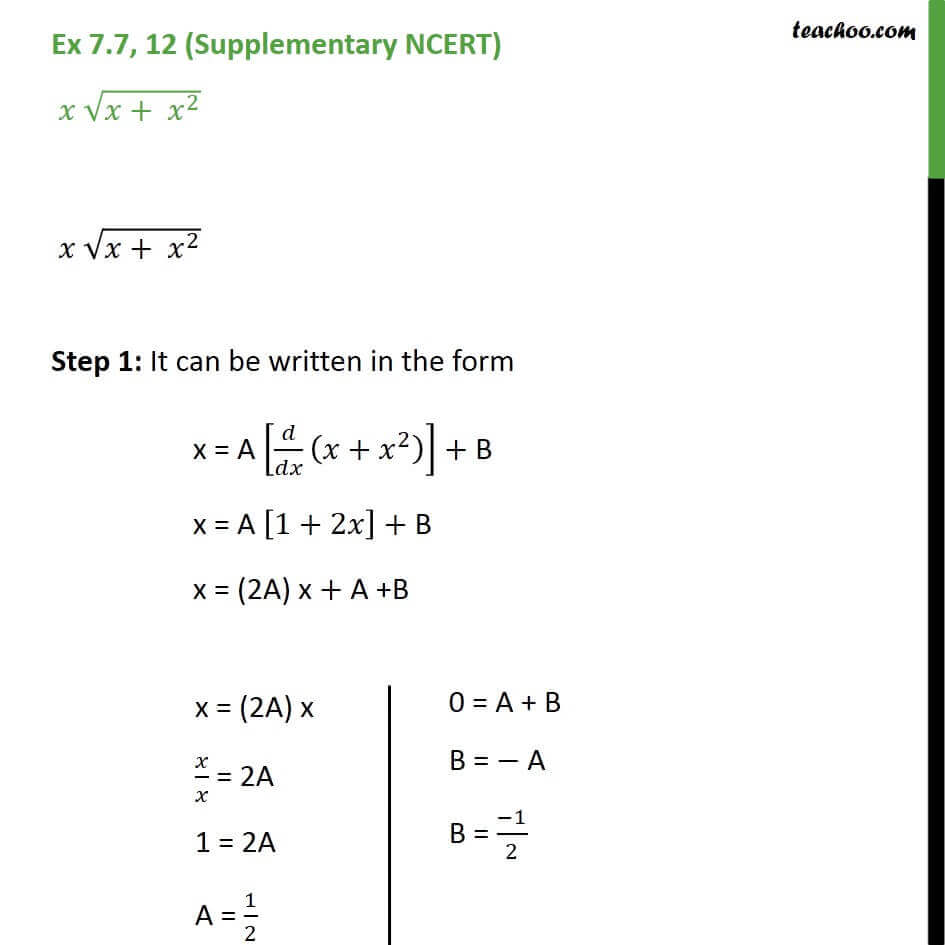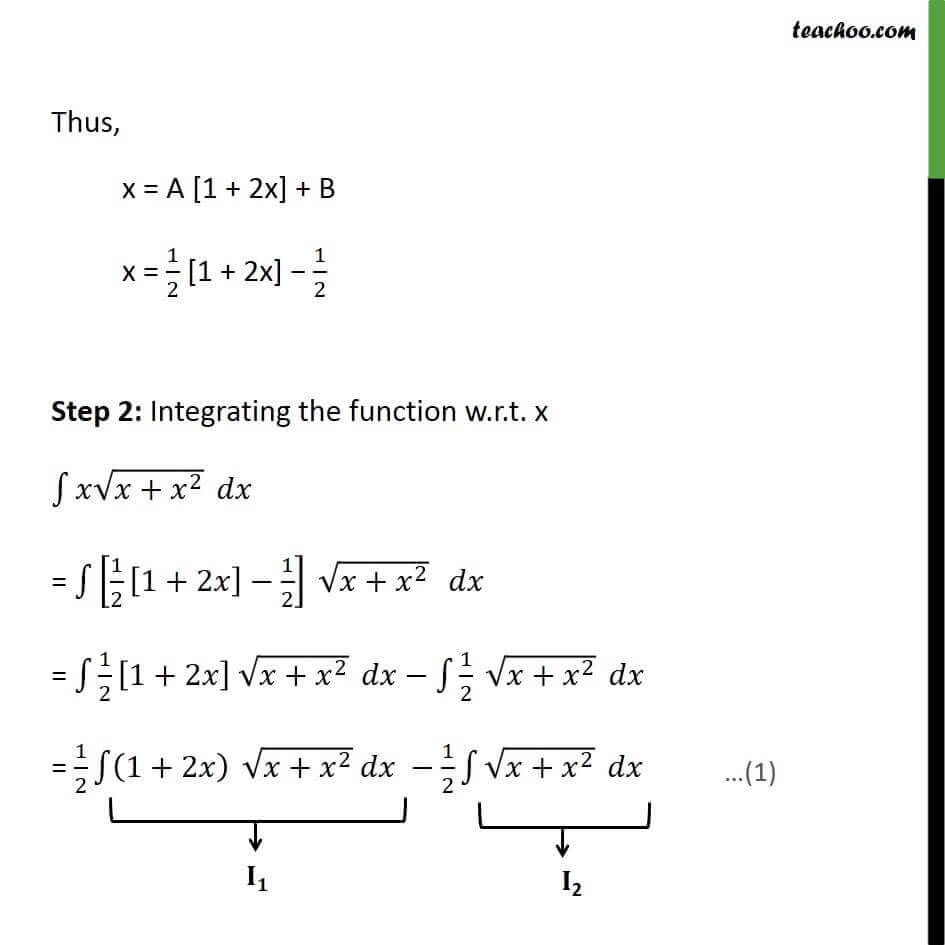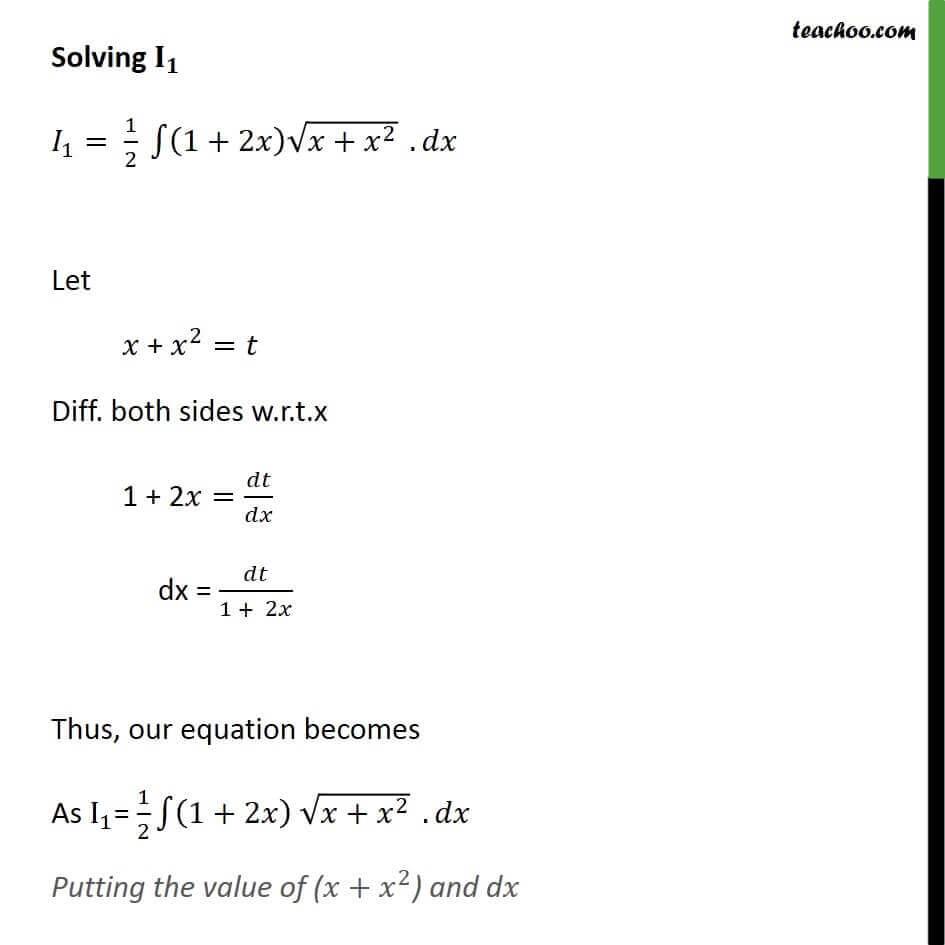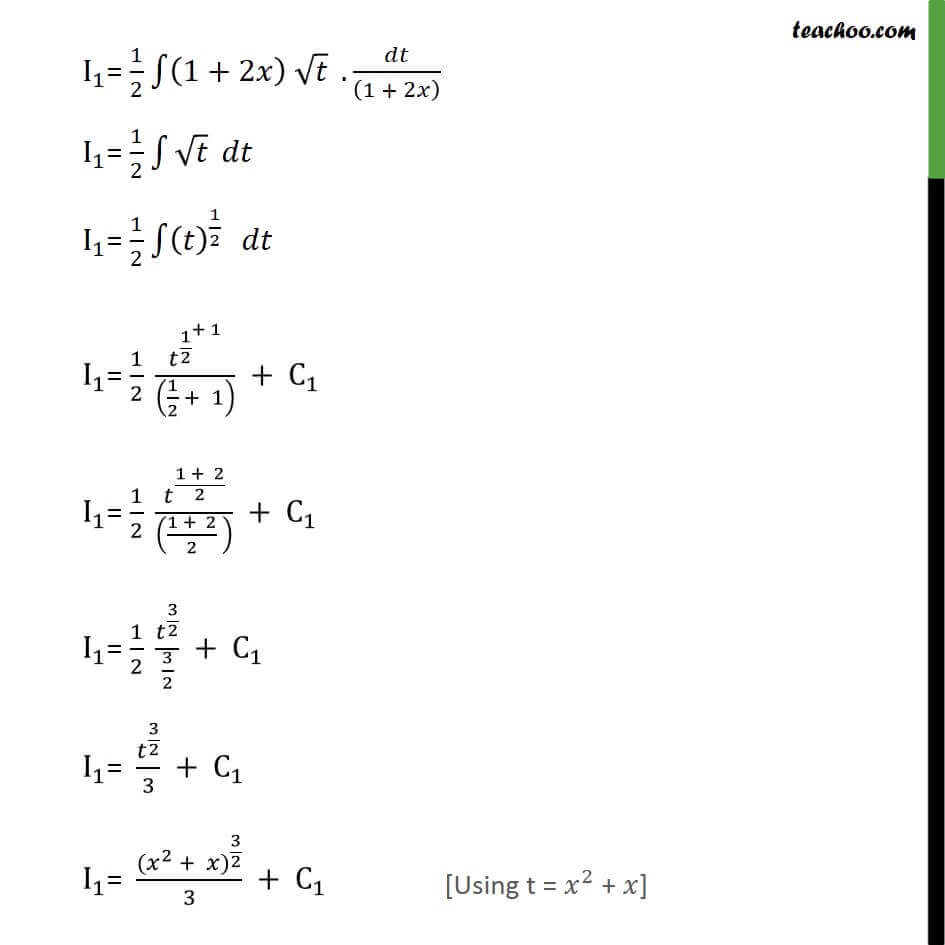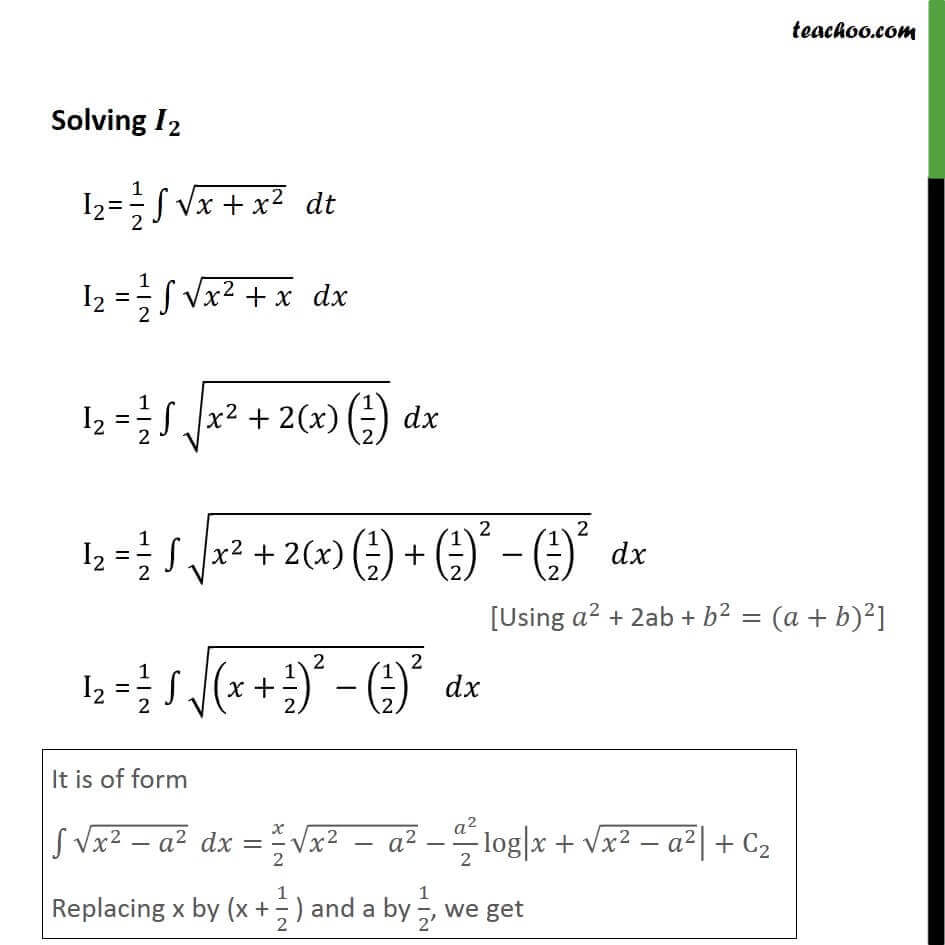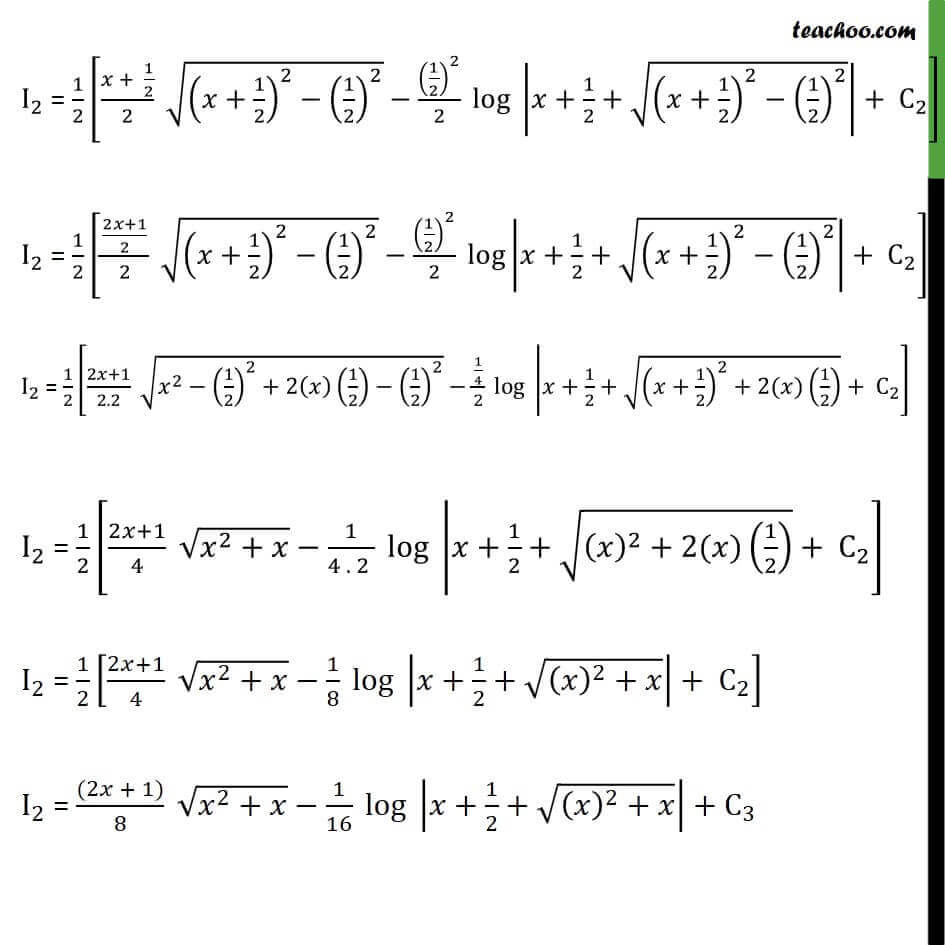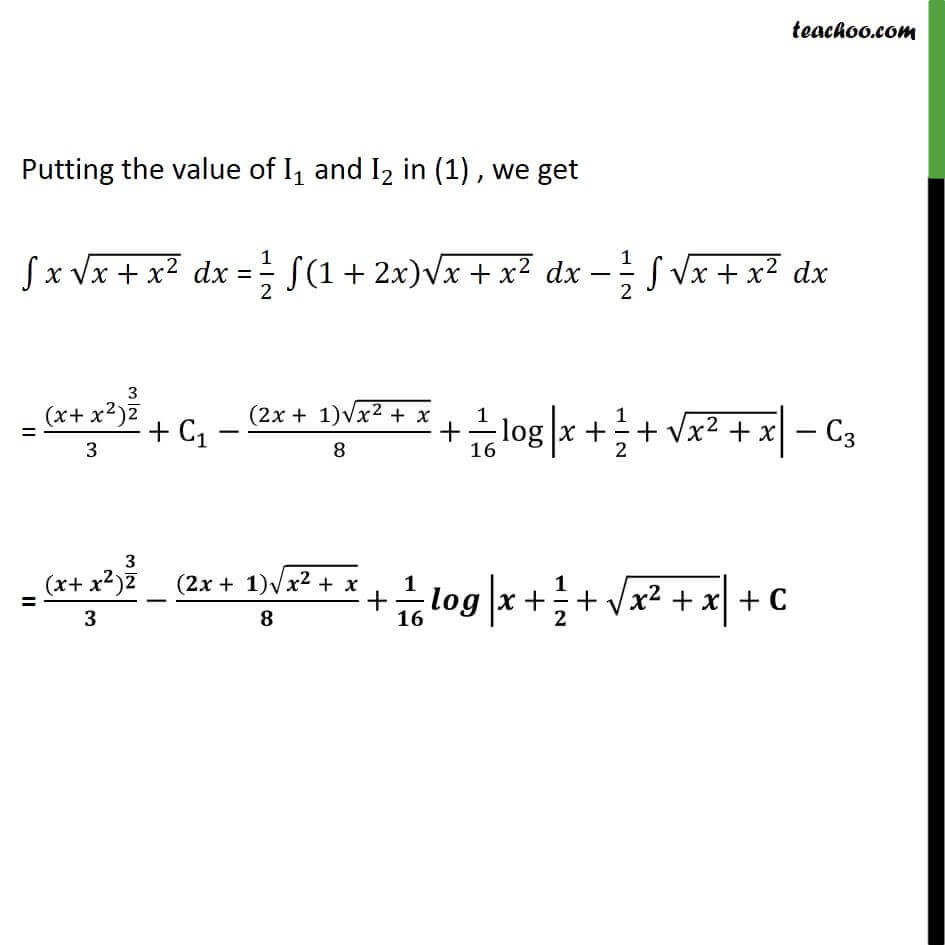Learn in your speed, with individual attention - Teachoo Maths 1-on-1 Class

### Transcript

Ex 7.7, 12 (Supplementary NCERT) ( + ^2 ) ( + ^2 ) Step 1: It can be written in the form x = A [ / ( + ^2 )]+ B x = A [1+2 ]+ B x = (2A) x + A +B x = (2A) x / = 2A 1 = 2A A = 1/20 = A + B B = A B = ( 1)/2 Thus, x = A [1 + 2x] + B x = 1/2 [1 + 2x] 1/2 Step 2: Integrating the function w.r.t. x 1 ( + ^2 ) = 1 [1/2 [1+2 ] 1/2] ( + ^2 ) = 1 1/2 [1+2 ] ( + ^2 ) 1 1/2 ( + ^2 ) = 1/2 1 (1+2 ) ( + ^2 ) 1/2 1 ( + ^2 ) Solving _ _1= 1/2 1 (1+2 ) ( + ^2 ) . Let + ^2= Diff. both sides w.r.t.x 1 + 2 = / dx = /(1 + 2 ) Thus, our equation becomes As I_1= 1/2 1 (1+2 ) ( + ^2 ) . Putting the value of ( + ^2) and d I_1= 1/2 1 (1+2 ) . /((1 + 2 ) ) I_1= 1/2 1 I_1= 1/2 1 ( )^(1/2) I_1= 1/2 ^( 1/2 ^(+ 1) )/((1/2 + 1) ) + C_1 I_1= 1/2 ^((1 + 2)/2)/(((1 + 2)/2 ) ) + C_1 I_1= 1/2 ^(3/2)/(3/2) + C_1 I_1= ^(3/2)/3 + C_1 I_1= ( ^2 + ) ^(3/2)/3 + C_1 Solving _ I_2= 1/2 1 ( + ^2 ) I_2 = 1/2 1 ( ^2+ ) I_2 = 1/2 1 ( ^2+2( )(1/2) ) I_2 = 1/2 1 ( ^2+2( )(1/2)+(1/2)^2 (1/2)^2 ) I_2 = 1/2 1 (( +1/2)^2 (1/2)^2 ) It is of form 1 ( ^2 ^2 ) = /2 ( ^2 ^2 ) ^2/2 log | + ( ^2 ^2 )|+C_2 Replacing x by (x + 1/2 ) and a by 1/2, we get I_2 = 1/2 [( + 1/2)/2 (( +1/2)^2 (1/2)^2 ) (1/2)^2/2 log | +1/2+ (( +1/2)^2 (1/2)^2 )|+ C_2 ] I_2 = 1/2 [((2 +1)/2)/2 (( +1/2)^2 (1/2)^2 ) (1/2)^2/2 log| +1/2+ (( +1/2)^2 (1/2)^2 )|+ C_2 ] I_2 = 1/2 [(2 +1)/2.2 ( ^2 (1/2)^2+2( )(1/2) (1/2)^2 ) (1/4)/2 log | +1/2+ (( +1/2)^2+2( )(1/2) ) + C_2 ] I_2 = 1/2 [(2 +1)/4 ( ^2+ ) 1/(4 . 2 ) log | +1/2+ (( )^2+2( )(1/2) ) + C_2 ] I_2 = 1/2 [(2 +1)/4 ( ^2+ ) 1/8 log | +1/2+ (( )^2+ )|+ C_2 ] I_2 = ((2 + 1))/8 ( ^2+ ) 1/16 log | +1/2+ (( )^2+ )|+C_3 Putting the value of I_1 and I_2 in (1) , we get 1 ( + ^2 ) = 1/2 1 (1+2 ) ( + ^2 ) 1/2 1 ( + ^2 ) = ( + ^2) ^(3/2)/3+C_1 ((2 + 1) ( ^2 + ))/8+1/16 log | +1/2+ ( ^2+ )| C_3 = ( + ^ ) ^( / )/ (( + ) ( ^ + ))/ + / | + / + ( ^ + )|+